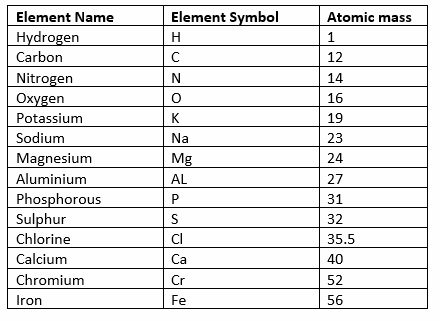# Numericals Problems for Atoms and Molecules

Given below are the Class 9 Science CBSE Numericals Problems for Atoms and Molecules
(a) Short questions
Here is the atomic mass of the common elementsHope you like them and do not forget to like , social share and comment at the end of the page.

Question 1
Convert into mole:
(i)12 g of oxygen gas
(ii)22 g of carbon dioxide

Question 2
How many moles of AI atoms are needed to combine with 1.58 moles of O atoms to make aluminum oxide, AI2O3?

Question 3
What is the volume of 32 g of sulphur dioxide measured at STP?

Question 4
How many grams of sodium will have the same number of atoms as 6 grams of magnesium? (Na = 23, Mg = 24).

Question 5
How many grams of Chromium (molar mass Cr = 52 g/mol) are there in 85 g of Cr2S3?

Question 6
Which has more number of atoms: 100 grams of sodium or 100 grams of iron (Given atomic mass of Na = 23 u and Fe = 56 u)

Question 7
A compound of nitrogen and oxygen has the formula NO. In this compound, there are 1.143 g of oxygen for each 1.000g of nitrogen. A different compound of nitrogen and oxygen has the formula NO2. How many grams of oxygen would be combined with each 1.000g of nitrogen in NO2?

Question 8
How many moles of nitrogen atoms are combined with 8.60 mol of oxygen atoms in dinitrogen pentoxide, N2O5
Question 9
Calculate the volume occupied by 2.8 g of N2 at STP.

Question 10
How much weight of carbon dioxide is present in same volume which is occupied by 4.0 grams of oxygen? Volumes are measured at normal temperature and pressure. (NTP)
Question 11
What is the mass of 0.5 mole of water (H2O). (Atomic masses: H = 1u, O = 16u)

Question 12
How many moles of sulphur atoms and oxygen atoms are present in one mole each of H2SO4, H2O3 and SO2?
Question 13
What is the percentage composition of the elements in ammonia, NH3? (at. Mass: H = 1, = 14)
Question 14
Calculate the theoretical percentage composition of N2O3.
Question 15
10 gram of CaCO3 on heating gave 4.4g of CO2 and 5.6g of CaO. Show that these observations are in agreement with the law of conservation.
Question 16
In which of the following, the number of hydrogen atoms is more:
(a) 3 moles of H2O
(b)10 moles of HCI

Question 17
If we decompose 50g of water by passing electricity through it, how many grams of oxygen and hydrogen we obtain?
Question 18
Hydrogen and oxygen combine I the ratio of 1: 8 by mass to form water. What mass of oxygen gas would be required to react completely with 3g of hydrogen gas?
Question 19
Calculate the number of molecules in 32 grams of oxygen gas and 14 grams of nitrogen.
Question 20
Calculate the mass of a sample of iron metal that would contain 0.250 moles of iron atoms.
Question 21
Calculate the number of atoms in a 0.123-gram sample of aluminium foil.

Question 1 Calculate the formula mass of each of the following and round your answer to the nearest 0.1 u.
(a) NaHCO3
(b) K2Cr2O7
(c) (NH4)2CO3
(d) AI2 (SO4)3
(e) CuSO4.5H2O
Question 2
Calculate the molar mass of the following substances.
(a)Ethyne, C2H2
(b)Sulphur molecule, S8
(c)Phosphorus molecule, P4 (atomic mass of phosphorus = 31)
(d)Hydrochloric acid, HCI
(e)Nitric acid, HNO3
Question 3
Calculate the number of aluminium ions present in 0.051 g of aluminium oxide (AI2O3).
(Atomic mass: AI = 27 u, O = 16 u)
Question 4
(i) Calculate both the average mass of a single molecule of carbon dioxide and glucose and the molecular weight of these compounds.
(ii) Determine the number of carbon atoms in 0.500 grams of carbon dioxide, CO2.
Question 5
(i) 1.375g of cupric oxide was reduced by heating in a current of hydrogen and the weight of copper that remained was 1.098g. in another experiment 1.179g of copper was dissolved in nitric acid and resulting copper nitrate converted into cupric oxide by ignition. The weight of cupric oxide formed was 1.476g. Show that these results illustrate the law of constant proportion.
(ii) in an experiment, 1.288 g of copper oxide was obtained from 1.03 g of copper. In another experiment, 3.672 g of copper oxide gave, on reduction, 2.938 g of copper. Show that these figures verify the law of constant proportions.
Question 6
Calculate the following quantities:
(a)Mass, in grams 1.73 mol CaH2
(b)Moles of Mg (NO3)2 in 3.25 g of this substance
(c)Number of molecules in 0.245 mol CH3OH
(d)Number of H atoms in 0.585 mol C4H10.
(e)Number of moles of AI are in 2.16 mol of AI2O3

## Summary

This Numericals Problems for Atoms and Molecules is prepared keeping in mind the latest syllabus of CBSE . This has been designed in a way to improve the academic performance of the students. If you find mistakes , please do provide the feedback on the mail.